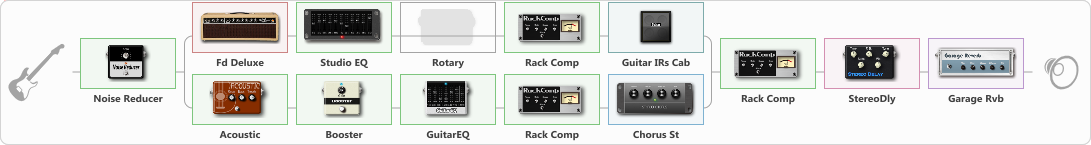# Timotei

Discussion in 'ToneLib-GFX presets' started by TimoteiURAL, May 2, 2021.

1. TDG Clean
Preset name: TDG Clean

Effects chain:Effect: "Noise Reducer" (Dynamics / Filter), active - "yes"
{

"Sens" = 38
"Mode" = Hard
}

Effect: "Splitter" (Dynamics / Filter)
{

"A-Bypass" = Off
"A-Pan" = 0
"A-Level" = 55
"B-Bypass" = Off
"B-Pan" = 0
"B-Level" = 49
'A' branch:
{

Effect: "Fd Deluxe" (Amp simulators), active - "yes"
{

"Gain" = 60
"Bass" = 28
"Middle" = 16
"Treble" = 57
"Presence" = 61
"Master" = 50
"Level (dB)" = 0
}
Effect: "Studio EQ" (Dynamics / Filter), active - "yes"
{

"31 Hz" = -15
"62 Hz" = -15
"125 Hz" = 2
"250 Hz" = 3
"500 Hz" = -4
"1 kHz" = 4
"2 kHz" = 2
"4 kHz" = 10
"8 kHz" = 14
"16 kHz" = 15
"Level (dB)" = 0
}
Effect: "Rotary" (Modulation / Sfx), active - "no"
{

"Speed A" = 10.0
"Speed B" = 10.0
"SpeedSw" = A
"Depth" = 43
"Accel" = 9.2
}
Effect: "Rack Comp" (Dynamics / Filter), active - "yes"
{

"Threshold (dB)" = -13
"Ratio" = 5
"Attack" = Fast
"Release (ms)" = 250
"Knee" = 25
"Level (dB)" = 0
}
Effect: "Guitar IRs Cab" (Cabinets), active - "yes"
{

"Model" = Fender Deluxe Rev (1x12")
"Mic Position" = Center
"Mic Distance" = Far
"Low Cut (Hz)" = 101
"Hi Cut (kHz)" = 10.0
"Mix" = 100
"Level (dB)" = 9
}
}
'B' branch:
{

Effect: "Acoustic" (Dynamics / Filter), active - "yes"
{

"Style" = Country/Blues
"Resonance" = 51
"Bass" = 6
"Treble" = 76
}
Effect: "Booster" (Dynamics / Filter), active - "yes"
{

"Gain" = 100
}
Effect: "GuitarEQ" (Dynamics / Filter), active - "yes"
{

"160 Hz" = -15
"400 Hz" = -8
"800 Hz" = -12
"1.6 kHz" = 9
"3.2 kHz" = -12
"6.4 kHz" = 5
"12 kHz" = -15
"Level (dB)" = 10
}
Effect: "Rack Comp" (Dynamics / Filter), active - "yes"
{

"Threshold (dB)" = -13
"Ratio" = 5
"Attack" = Fast
"Release (ms)" = 250
"Knee" = 25
"Level (dB)" = 0
}
Effect: "Chorus St" (Modulation / Sfx), active - "yes"
{

"Speed" = 0.1
"Depth" = 0
"Center" = 5.4
"Mix" = 100
}
}
}

Effect: "Rack Comp" (Dynamics / Filter), active - "yes"
{

"Threshold (dB)" = -8
"Ratio" = 5
"Attack" = Fast
"Release (ms)" = 250
"Knee" = 25
"Level (dB)" = 0
}

Effect: "StereoDly" (Delay), active - "yes"
{

"Time" = 333
"Feedback" = 44
"Tone" = 12
"Sens" = 100
"Mix" = 32
}

Effect: "Garage Rvb" (Reverberation), active - "yes"
{

"Time" = 4.6
"PreDelay" = 20
"LoDamp" = 0
"HiDamp" = 18
"Mix" = 39
}

Note: You will need to download and install the ToneLib-GFX software to use the preset.

#### Attached Files:

• ###### Timotei.tlgfx
File size:
1.4 KB
Views:
2,513
Last edited: May 2, 2021
André Ezías likes this.